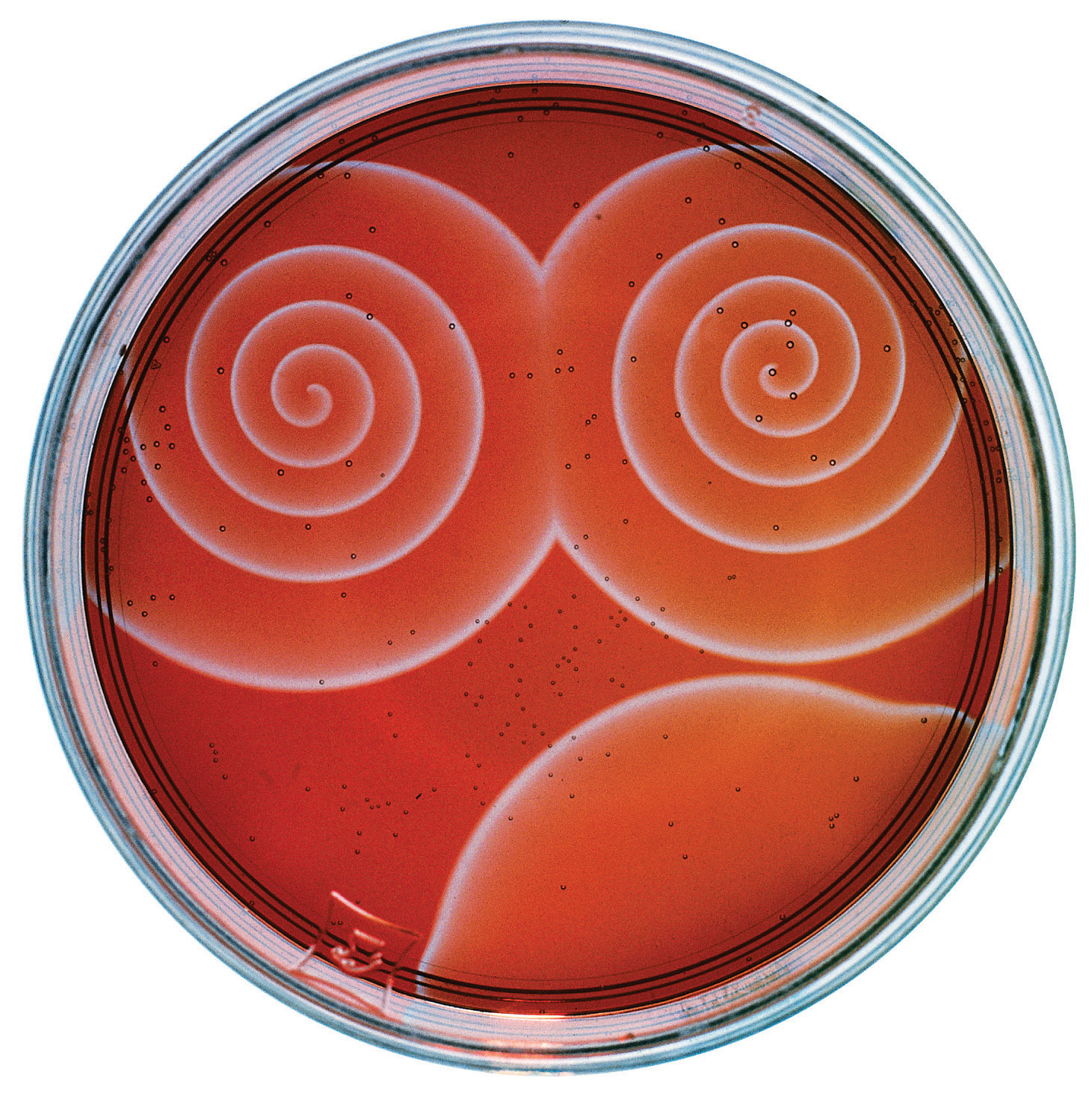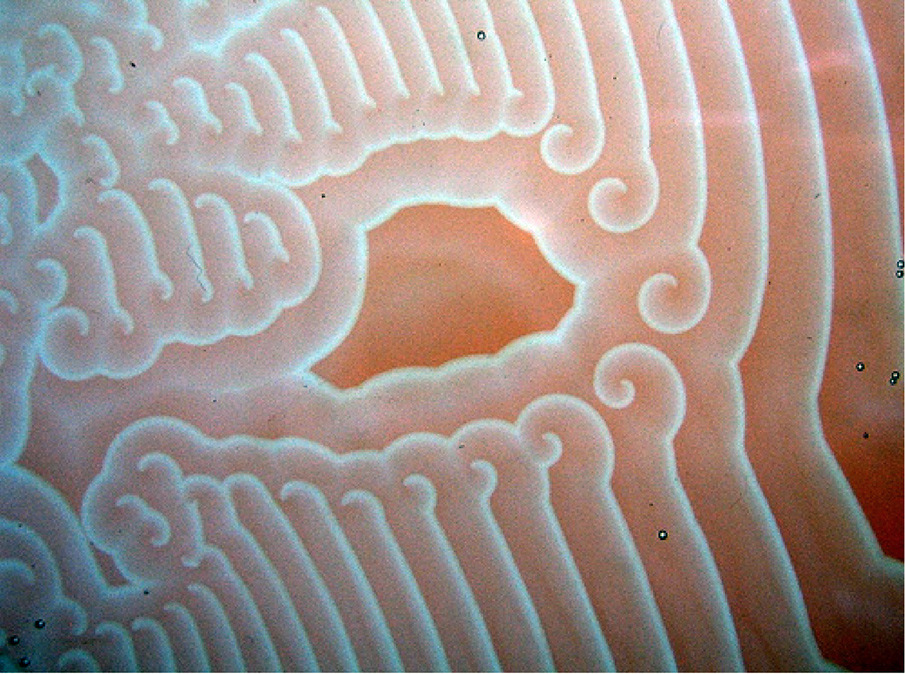# Fractal Spirographs

Inspiration

Daniel Shiffman has been making YouTube movies for some time now. His videos focus on programming and include coding challenges in which he writes code for a target idea from scratch. If you are a coder I recommend Dan’s videos for entertainment and inspiration.

His latest live stream focused on Fractal Spirographs.

If you prefer to watch a shorter edited version, here it is.

He was inspired by the following image from the Benice Equation blog.Fractal Spirographs (aka Fractal Routlette) are generated by tracking a series (or chain) of circles rotating around each other as shown in the above gif animation. You track the chain of 10 or so circles and plot the path the final smallest circle takes. Changing the number of circles, the size ratio between circles, the speed of angle change, and the constant “k” changes the resulting plots and images.

How I Coded It

As I watched Daniel’s video I coded my own version. For my code (Delphi/pascal) I used a dynamic array of records to hold the details of each circle/orbit. This seemed the simplest approach to me for keeping track of a list of the linked circles.

``````
type orbit=record
x,y:double;
angle:double;
speed:double;
end;
```
```

Before the main loop you fill the array;

``````
//parent orbit
orbits.x:=destimage.width/2;
orbits.y:=min(destimage.width,destimage.height)/2;
orbits.angle:=0;
orbits.speed:=0;
//children orbits
for loop:=1 to numorbits-1 do
begin
newy:=orbits[loop-1].y;
orbits[loop].x:=newx;
orbits[loop].y:=newy;
orbits[loop].angle:=orbits[loop-1].angle;
orbits[loop].speed:=power(k,loop-1)/sqr(k*k);
end;
```
```

Then inside the main loop, you update the orbits;

``````
//update orbits
for loop:=1 to numorbits-1 do
begin
orbits[loop].angle:=orbits[loop].angle+orbits[loop].speed;
orbits[loop].x:=orbits[loop-1].x+rsum*cos(orbits[loop].angle*pi/180);
orbits[loop].y:=orbits[loop-1].y+rsum*sin(orbits[loop].angle*pi/180);
end;
```
```

and then you use the last orbit positions to plot the line, ie

``````
canvas.lineto(round(orbits[numorbits-1].x),round(orbits[numorbits-1].y));
```
```

Results

Once the code was working I rendered the following images and movie. They are all 4K resolution to see the details. Click the images to see them full size.Here is a 4K movie showing how these curves are built up.

Fractal Spirographs are now included with the latest version of Visions of Chaos.

Finally, here is an 8K Fulldome 8192×8192 pixel resolution image. Must be seen full size to see the fine detailed plot line.To Do

Experiment with more changes in the circle sizes. The original blog post links to another 4 posts here, here, here and here and even this sumo wrestlerPlenty of inspiration for future enhancements.

I have already experimented with 3D Spirographs in the past, but they are using spheres rotating within other spheres. Plotting the sqheres rotating around the outside of other spheres should give more new unique results.

Jason.

# The Burning Ship Fractal

The Burning Ship Fractal is a slight variant on the Mandelbrot Set Fractal.

The basic Mandelbrot Fractal formula is z=z^2+c. The Burning Ship Fractal formula is z=abs(z)^2+c.

The following image is the standard power 2 Burning Ship Fractal rendered using CPM smooth coloring.Zooming in to the right antenna part of the fractal shows why it was named the Burning Ship.The next 3 images change the exponent 2 in z=abs(z)^2+c to 3, 4 and 5.The same power 2 through power 5 Burning Ships but this time using Triangle Inequality Average (TIA) coloringThe next 4K resolution movie shows a series of zooms into Burning Ship Fractals between power 2 and power 5 colored using CPM coloring

and finally another 4K movie showing more Burning Ship zooms colored using TIA coloring

All of the above images and movies were created with Visions of Chaos.

Jason.

# Meta-Mandelbrots

Ian McDonald came up with a new novel way to render Mandelbrot (actually Julia) Set fractals.

The usual Mandelbrot fomula is
z=z*z+c

Taking the z*z+c part, replace the z’s with (z*z+c) and replace the c’s with (c*c+z)

After one level of replacement you get
((z*z+c)*(z*z+c)+(c*c+z))

Level 2 is
(((z*z+c)*(z*z+c)+(c*c+z)) * ((z*z+c)*(z*z+c)+(c*c+z)) + ((c*c+z)*(c*c+z)+(z*z+c)))

and Level 3 is
((((z*z+c)*(z*z+c)+(c*c+z))*((z*z+c)*(z*z+c)+(c*c+z))+((c*c+z)*(c*c+z)+(z*z+c)))*(((z*z+c)*(z*z+c)+(c*c+z))*((z*z+c)*(z*z+c)+(c*c+z))+((c*c+z)*(c*c+z)+(z*z+c)))+(((c*c+z)*(c*c+z)+(z*z+c))*((c*c+z)*(c*c+z)+(z*z+c))+((z*z+c)*(z*z+c)+(c*c+z))))

Then you use the level 3 formula and render it as a Julia Set.

Complex C (-0.2,0.0)Complex C (-0.14 0.0)Complex C (-0.141 0.0)The following movie shows the complex C changing slowly from 0 to -0.2 and three zooms into Meta-Mandelbrots. Unfortunately because these are Julia sets the shapes deeper in are virtually identical to the original fractal. You don’t get those totally different looking areas as you do with Mandelbrot fractals.

The GLSL shader to generate these fractals is now included with Visions of Chaos.

# The Belousov-Zhabotinsky Reaction and The Hodgepodge Machine

Inspiration for this post

The other day I saw this YouTube video of a Belousov-Zhabotinsky Cellular Automaton (BZ CA) by John BitSavage

After a while of running in an oscillating state it begins to grow cell like structures. I had never seen this in BZ CAs before. I have seen similar cell like growths in Digital Inkblot CAs and in the Yin Yang Fire CA. Seeing John’s results different to the usual BZ CA was what got me back into researching BZ in more depth.

The Belousov-Zhabotinsky Reaction

Belousov-Zhabotinsky Reactions (see here for more info) are examples of a chemical reactions that can oscillate between two different states and form intetesting patterns when performed in shallow petri dishes.

Here are some sample high res images of real BZ reaction by Stephen Morris. Click for full size.and some other images from around the Internetand some sample movies I found on YouTube

The Hodgepodge Machine Cellular Automaton

Back in August 1988, Scientific American‘s Computer Recreations section had an article by A. K. Dewdney named “The hodgepodge machine makes waves”. After a fair bit of hunting around I could not find any copies of the article online so I ended up paying \$8 USD to get the issue in PDF format. The PDF is a high quality version of the issue, but \$8 is still a rip off.

In the article Dewdney describes the “hodgepodge machine” cellular automaton designed by Martin Gerhardt and Heike Schuster of the University of Bielefeld in West Germany. A copy of their original paper can be seen here.

How the Hodgepodge Machine works

The inidividual cells/pixels in the hodgepodge automaton have n+1 states (between 0 and n). Cells at state 0 are considered “healthy” and cells at the maximum state n are said to be “ill”. All cells with states inbetween 0 and n are “infected” with the larger the state representing the greater level of infection.

Each cycle of the cellular automaton a series of rules are applied to each cell depending on its state.

(a) If the cell is healthy (i.e., in state 0) then its new state is [a/k1] + [b/k2], where a is the number of infected cells among its eight neighbors, b is the number of ill cells among its neighbors, and k1 and k2 are constants. Here “[]” means the integer part of the number enclosed, so that, for example, [7/3] = [2+1/3] = 2.

(b) If the cell is ill (i.e., in state n) then it miraculously becomes healthy (i.e., its state becomes 0).

(c) If the cell is infected (i.e., in a state other than 0 and n) then its new state is [s/(a+b+1)] + g, where a and b are as above, s is the sum of the states of the cell and of its neighbors and g is a constant.

The parameters given for these CA are usual q (for max states), k1 and k2 (the above constants) and g (which is a constant for how quickly the infection tends to spread).

My previous limited history experimenting with BZ

Years ago I implemented BZ CA in Visions of Chaos (I have now correctly renamed the mode Hodgepodge Machine) and got the following result. This resolution used to be considered the norm for YouTube and looked OK on lower resolution screens. How times have changed.

The above run used these parameters
q=200
k1=3
k2=3
g=28

Replicating Gerhardt and Miner’s results

Gerhadt and Miner used fixed values of k1=2 and k2=3. The majority of their experiments used a grid size of q=20 (ie only 20×20 cells) without a wraparound toroidal world. This leaves the single infection spreading g variable to play with. Their paper states they used values of g between 1 and 10, but I get no spirals with g in that range.

Here are a few samples which are 512×512 sized grids with wraparound edges and many thousands of generations to be sure they had finally settled down. Each cell is 2×2 pixels in size so they are 1024×1024 images.q=100, k1=2, k2=3, g=5q=100, k1=2, k2=3, g=20q=100, k1=2, k2=3, g=25q=100, k1=2, k2=3, g=30

Results from other parametersq=100, k1=3, k2=3, g=10q=100, k1=3, k2=3, g=15q=100, k1=3, k2=3, g=20

Extending into 3D

The next logical step was extending it into three dimensions. This blog post from Rudy Rucker shows a 3D BZ CA from Harry Fu back in 2004 for his Master’s degree writing project. I must be a nerd as I whipped up my 3D version over two afternoons. Surprisingly there are no other references to experiments with 3D Hodgepodge that I can find.

The algorithms are almost identical to their 2D counterparts. The Moore neighborhood is extended into three dimensions (so 26 neighbors rather than 8 in the 2D version). It is difficult to see the internal structures as they are hidden from view. Methods I have used to try and see more of the internals are to slice out 1/8th of the cubes and to render only some of the states.

Clicking these sample images will show them in 4K resolution.q=100, k1=1, k2=18, g=43 (150x150x150 grid)q=100, k1=1, k2=18, g=43 (150x150x150 grid – same as previous with a 1/8th slice out to see the same patterns are extending through the 3D structure)q=100, k1=1, k2=18, g=43 (150x150x150 grid – same rules again, but this time with only state 0 to state 50 cells being shown)q=100, k1=2, k2=3, g=15 (150x150x150 grid)q=100, k1=3, k2=6, g=31 (150x150x150 grid)q=100, k1=4, k2=6, g=10 (150x150x150 grid)q=100, k1=4, k2=6, g=10 (150x150x150 grid – same rules as the previous image – without the 1/8th slice – with only states 70 to 100 visible)q=100, k1=3, k2=31, g=43 (250x250x250 sized grid – 15,625,000 total cells)q=100, k1=4, k2=12, g=34 (350x350x350 sized grid – 42,875,000 total cells)q=100, k1=1, k2=9, g=36 (400x400x400 sized grid – 64,000,000 total cells)

Download Visions of Chaos if you would like to experiment with both 2D and 3D Hodgepodge Machine cellular automata. If you find any interesting rules please let know in the comments or via email.